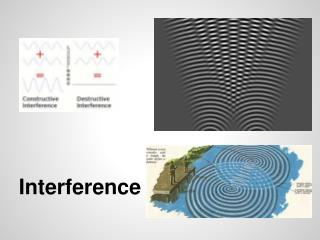DownloadDownload PresentationInterference

# Interference

Download Presentation## Interference

- - - - - - - - - - - - - - - - - - - - - - - - - - - E N D - - - - - - - - - - - - - - - - - - - - - - - - - - -

1. ### Interference

2. Standing Waves A wave that appears to be standing still, produced by the interference of two traveling waves moving in opposite directions
3. Node – the stationary point where two equal wave pulses meet and are in the same location, have a displacement of zero Antinode – the point with the largest displacement when two wave pulses meet
5. ### Periodic Motion Pendulums & Springs

6. Periodic Motion Periodic Motion is an example of vibrational motion. In this motion, an object moves back and forth with some degree of predictability Ex. Time to go back and forth Distance traveled each time Examples include a clock pendulum, molecular vibrations and wave motion.
7. Periodic Motion Characteristics of periodic motion: Period (T) which is the time taken to complete one cycle. Amplitude - Distance from equilibrium that an object moves.
8. Amplitude
9. Period The Period (T)of a pendulum is related to the length of the pendulum and gravity (the Force that results in motion). T = period (s) L = Length (m) g = 9.8m/s2
10. Pendulum Lab
11. Pendulum Video (minute 42ff)
12. Calculating Period Calculate the time it takes a 26cm pendulum to make 5 cycles.
13. A pendulum with a length of 36.9cm has a period of 1.22s. What is the acceleration due to gravity at the pendulum’s location? 9.78 m/s2
14. Hooke's Law A spring is a device that stores potential energy. When a spring is stretched (or compressed), a force is applied through a distance. Thus, work is done. The work done goes to the spring in the form of potential energy (EP). The spring force is a restoring force which, in bringing the spring back to its rest point, does work. Note that every spring has an elastic limit. If the spring is stretched Within its elastic limit, it “springs” back to its “rest point”. If a spring is stretched beyond its elastic limit, it becomes deformed. Click
15. Hooke's Law As illustrated below, the distance a spring is stretched is called the “elongation”. Robert Hooke was the first to discover that the spring force is directly proportional to the elongation. Today, we call this law Hooke’s Law. The formula for the spring is: FS = kx (Hooke’s Law) Where: FS = the spring force (N) k = the spring constant (N/m) x = the elongation (m) Click
16. Hooke's Law The spring constant is different for different springs and depends upon the type of material the spring is made of as well as the thickness of the spring coil. The greater the value of the spring constant, the “stiffer” the spring.. The formula for the potential energy stored in a spring is: PE = ½ kx2 Where: EP = Potential energy (in joules) k = the spring constant (in N/m) x = the elongation (in meters) Remember that in an ideal spring, there is no loss of energy (EP) due to friction. Click
17. Hooke's Law When the spring force (FS) is plotted versus the elongation (x) of the spring, the resulting graph is a linear relation. The slope of the curve represents the spring constant while the area under the curve represents the potential energy stored in the spring. Click
18. A spring stretches by 18cm when a bag of potatoes weighing 56 N is suspended from its end. Determine the spring constant. How much elastic potential energy is stored in the spring when it is stretched this far? 310 N/m 5.0J